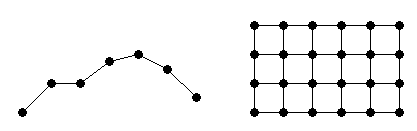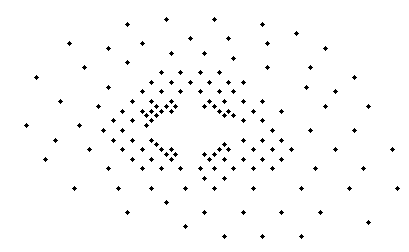Documentation

Gridded and Scattered Sample Data

Interpolation is a method for estimating the value at a query location that lies within the domain of a set of sample data points. A sample data set defined by locations X and corresponding values V can be interpolated to produce a function of the form V = F(X). This function can then be used to evaluate a query point Xq, to give Vq = F(Xq). This is a single-valued function; for any query Xq within the domain of X it will produce a unique value Vq. The sample data is assumed to respect this property in order to produce a satisfactory interpolation. One other interesting characteristic is that the interpolating function passes through the data points. This is an important distinction between interpolation and curve/surface fitting. In fitting, the function does not necessarily pass through the sample data points.

The computation of the value Vq is generally based on the data points in the neighborhood of the query point Xq. There are numerous approaches to performing interpolation. In MATLAB® interpolation is classified into two categories depending on the structure of the sample data. The sample data may be ordered in a axis-aligned grid or they may be scattered. In the case of a gridded distribution of sample points, you can leverage the organized structure of the data to efficiently find the sample points in the neighborhood of the query. Interpolation of scattered data on the other hand requires a triangulation of the data points, and this introduces an additional level of computation.

The two approaches to interpolation are covered in the following sections:

• The Interpolating Gridded Data section covers 1-D interpolation, and the N-D (N ≥ 2) interpolation of sample data in axis-aligned grid format:• The Interpolating Scattered Data section covers the N-D (N ≥ 2) interpolation of scattered data: